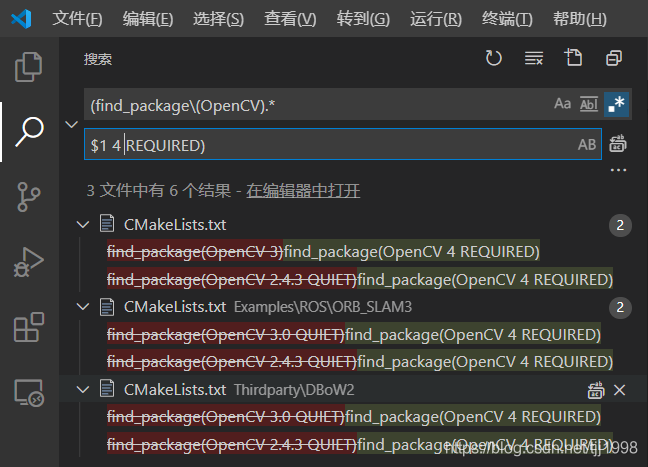# [solution] build vins and orb-slam based on opencv4

Scheme for regular substitution via VScode.When encountering an incorrect version of OpenCV

1. Find.

`(find_package\(OpenCV).*`

1. Replace:

`\$1 REQUIRED)`

1. CV_GRAY2RGB
1. Find:

`CV_(.*GRAY[^)]*)`

Replace:

`cv::COLOR_\$1`

1. CV_AA
1. Replace:

`CV_AA`

1. Replace:

`cv::LINE_AA`

1. CV_CALIB_*
1. Replace:

`CV_(CALIB[^ ,)]*)`

1. Replace:

`cv::\$1`

1. Find:

`CV_LOAD_IMAGE_([^ )]*)`

1. Replace:

`cv::IMREAD_\$1`

`#include<opencv/cv.h>`
``````#include <opencv2/imgproc/imgproc_c.h>